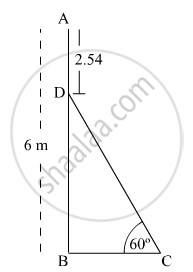# In Fig. 1, AB is a 6 m high pole and CD is a ladder inclined at an angle of 60° to the horizontal and reaches up to a point D of pole. If AD = 2.54 m, find the length of the ladder. - Mathematics

In Fig. 1, AB is a 6 m high pole and CD is a ladder inclined at an angle of 60° to the horizontal and reaches up to a point D of pole. If AD = 2.54 m, find the length of the ladder. (use3=1.73)#### Solution

In the given figure,

AB = AD + DB = 6 m

⇒ 2.54 m + DB = 6 m

⇒ DB = 3.46 m

Now, in the right triangle BCD,

(BD)/(CD)=sin 60^@

=>(3.46m)/(CD)=sqrt3/2

=>(3.46m)/(CD)=1.73/2

=>CD=(2xx3.46m)/1.73

CD=4 m

Thus, the length of the ladder CD is 4 m.

Concept: Heights and Distances
Is there an error in this question or solution?
2015-2016 (March) Delhi Set 1

Share